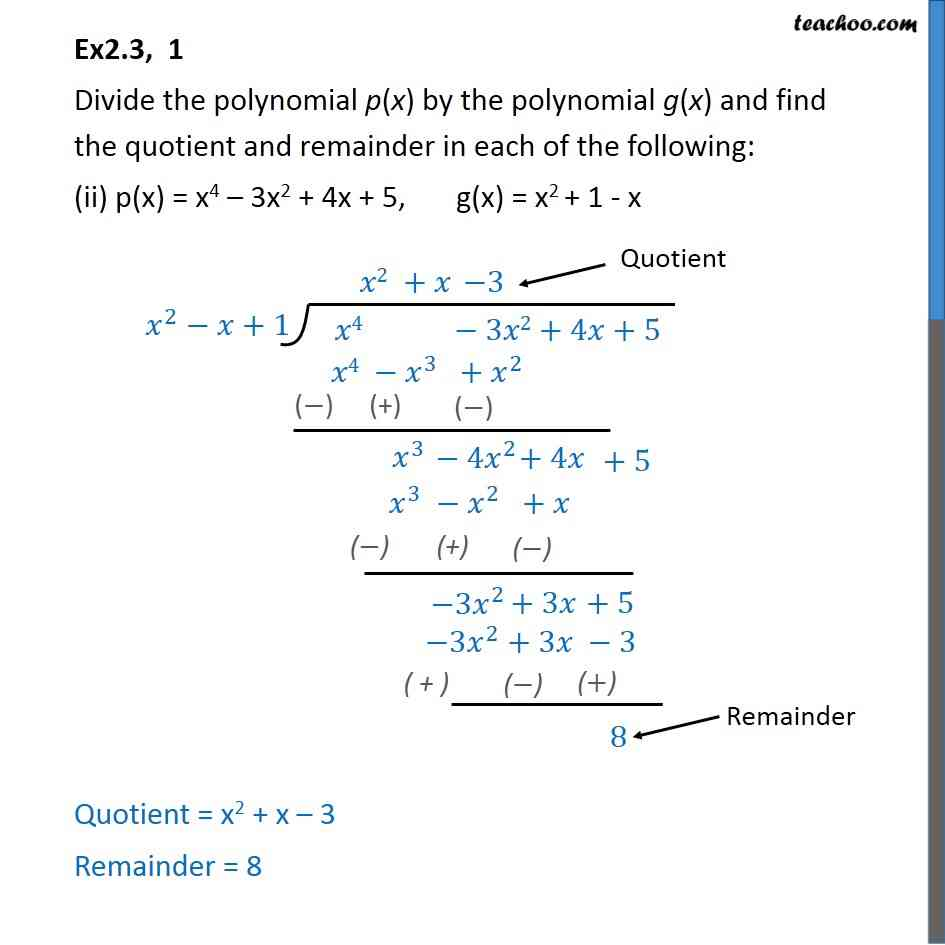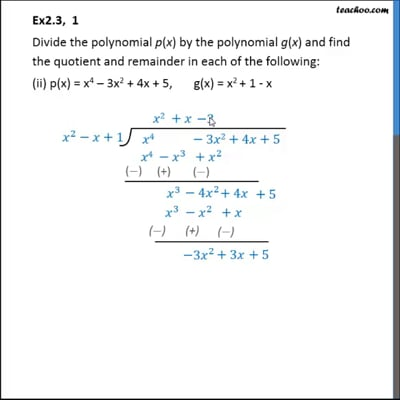Ex 2.3

Chapter 2 Class 10 Polynomials
Serial order wiseThis video is only available for Teachoo black users

Introducing your new favourite teacher - Teachoo Black, at only ₹83 per month

### Transcript

Ex2.3, 1 Divide the polynomial p(x) by the polynomial g(x) and find the quotient and remainder in each of the following: (ii) p(x) = x4 – 3x2 + 4x + 5, g(x) = x2 + 1 - x Quotient = x2 + x – 3 Remainder = 8